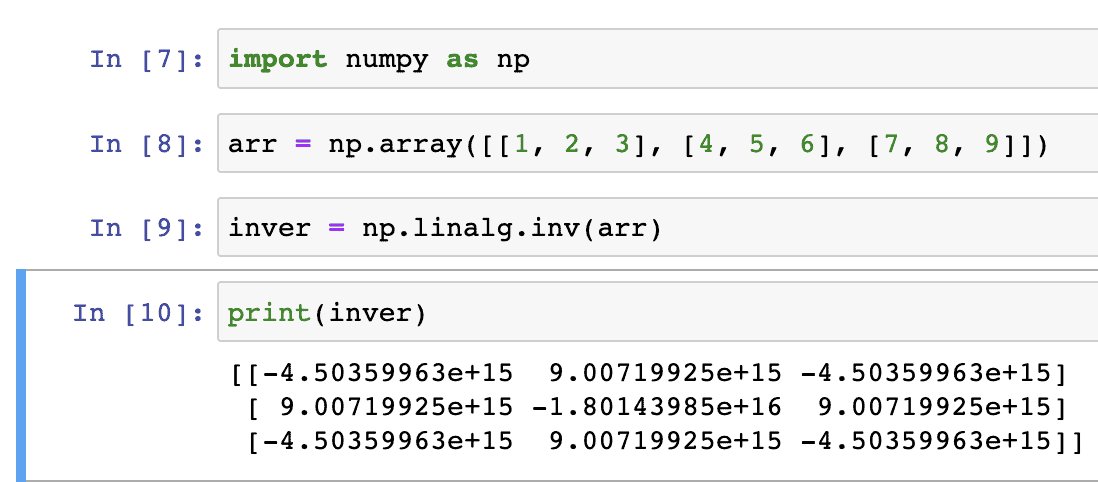# How to Fix LinAlgError: Last 2 dimensions of the array must be square

Python raises numpy.linalg.LinAlgError: Last 2 dimensions of the array must be square error when you try to perform a linear algebra operation on an array that doesn’t have the correct shape.

The main reason for the “linalgerror: last 2 dimensions of the array must be square” error is that the last two dimensions of the array must be square, meaning that the number of rows must be equal to the number of columns.

For example, if you have a 3×3 array, the last two dimensions are square, and you can perform linear algebra operations on it, but if you have a 3×4 array, the last two dimensions are not square, and you will receive this error message.

### Python code that generates a linalgerror

``````import numpy as np

# Creating a non-square matrix
a = np.array([[1, 2, 3], [4, 5, 6]])

# Attempting to perform a linear algebra operation
b = np.linalg.inv(a)
print(b)``````

OutputLinAlgError is an error that the NumPy library throws you when you try to do a linear algebra operation on a matrix that isn’t right. This error usually happens when the input matrix can’t be invertible or isn’t a square.

You can see that the above code raised a LinAlgError, with the message “linalgerror: last 2 dimensions of the array must be square”.

The error is raised because the inv() function, which calculates the inverse of a matrix, requires the input to be a square matrix, but a has shape (2, 3), so it is not square.

## How to Fix LinAlgError: Last 2 dimensions of the array must be square

You can fix the ” LinAlgError: Last 2 dimensions of the array must be square” error by ensuring that the input matrix is square.

``````import numpy as np

# Creating a square matrix
arr = np.array([[1, 2, 3], [4, 5, 6], [7, 8, 9]])

# Attempting to perform a linear algebra operation
inver = np.linalg.inv(arr)
print(inver)``````

OutputIn this code example, arr has shape (3, 3), so it is a square matrix, and you can perform linear algebra operations on it without encountering the error.

You can see that the error is resolved now, and we get the expected output.

This site uses Akismet to reduce spam. Learn how your comment data is processed.